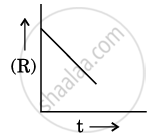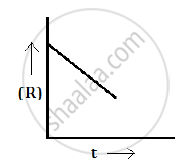Department of Pre-University Education, KarnatakaPUC Karnataka Science Class 12

# For a Chemical Reaction R → P, the Variation in the Concentration (R) Vs. Time (T) Plot is Given as - Chemistry

For a chemical reaction R → P, the variation in the concentration (R) vs. time (t) plot is given as:(i) Predict the order of the reaction.

(ii) What is the slope of the curve ?

(iii) Write the unit of rate constant for this reaction.

(iii) Write the unit of rate constant for this reaction.

#### Solution 1

For a chemical reaction R→P, the variation in the concentration (R) vs. time (t) plot is given as follows:(i) The order of the reaction zero.

(ii) Slope = -k

#### Solution 2

(i) The variation in the concentration (R) vs. time (t) plot shown here represents a zero order reaction, for which the rate of the reaction is proportional to zero power of the concentration of the reactants.

(ii) For a zero order reaction, rate constant is given as

k=([R]_0-[R])/t

So, the slope of the curve for the variation in the concentration (R) vs. time (t) plot is equal to the negative of the rate constant for the reaction.

#### Solution 3

(iii) Since it is a first order reaction, the unit of the rate constant is s−1.

Concept: Factors Influencing Rate of a Reaction
Is there an error in this question or solution?